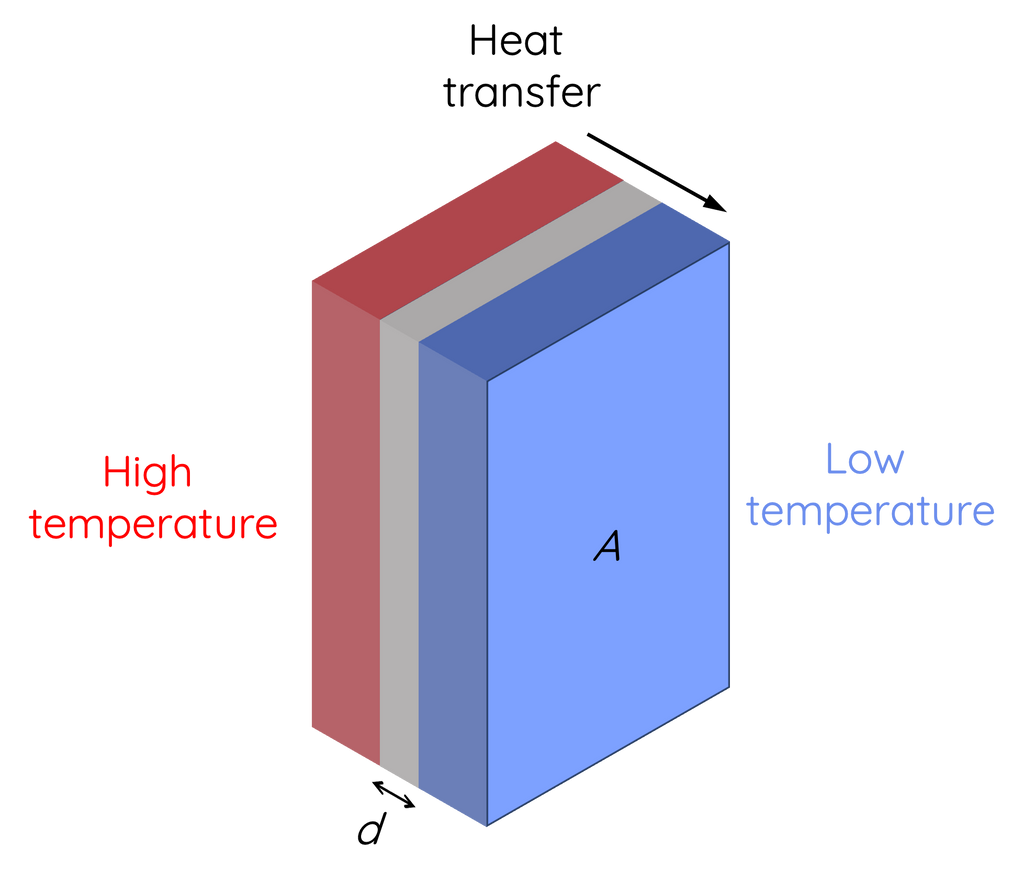# Thermal Conductivity

This topic is part of the HSC Physics course under the section Thermodynamics.

### HSC Physics Syllabus

• apply the following relationships to solve problems and make quantitative predictions in a variety of situations:
– Q=mc\DeltaT, where c is the specific heat capacity of a substance
– \frac{Q}{t} = \frac{kA \DeltaT}{d} where k is the thermal conductivity of a material

### What is Thermal Conductivity?

Thermal conductivity describes how effectively a material can conduct heat. In simple terms, it measures how quickly heat can travel through a substance. This property is especially significant in applications like building insulation, electronics cooling, and cooking utensils.

Materials that are good conductors of heat, like metals, have a high rate of heat transfer, while insulators, like wood or rubber, have a low rate. The ability of a material to conduct heat is quantified by its thermal conductivity k.The equation governing the rate of heat transfer due to conduction is:

$$\frac{Q}{t} = \frac{kA \Delta T}{d}$$

Where:

• Q/T is the rate of heat transfer (Unit: Watt or Joules per second).
• k is the thermal conductivity of the material (Unit: W m-1 K-1).
• A is the surface area through which heat is conducted (Unit: m2).
• \Delta T is the temperature difference across the material (Unit: K or ºC).
• d is the thickness of the material (Unit: m).

From the equation, it's evident that as the temperature difference \DeltaT decreases, the rate of heat transfer Q/t also decreases, assuming all other factors remain constant.

In other words, when there's a large temperature difference between two sides of a material, heat will flow more rapidly from the hotter side to the colder side. As the temperature difference decreases, this flow of heat will slow down. If there's no temperature difference (i.e., both sides are at thermal equilibrium), there will be no heat transfer through conduction.

### Example 1

A steel wall with a thermal conductivity k =  W m-1 K-1, a surface area of 2 m2, a thickness of  m, and a temperature difference across the wall °C. Calculate the initial rate of heat transfer through the wall.

Solution:

$$\frac{Q}{t} = \frac{kA \Delta T}{d}$$

$$\frac{Q}{t} = 50 \times 2 \times \frac{30}{0.01}$$

$$\frac{Q}{t} = 300,000 \, \text{W}$$

### Example 2

4.00 kg of ice is put in a cubical icebox of side 0.300 m has a thickness of 5.00 cm at 0ºC. Use the following information for this question.

• Temperature outside the box is constant at 45.0 ºC
• Coefficient of thermal conductivity of the ice box is 0.0100 W m–1 K–1
• Latent heat of fusion of water = 335 kJ kg–1.

Assume the temperature difference between inside and outside the icebox is constant. Estimate the mass of ice remaining after 6.00 hours.

Solution:

$$\frac{Q}{t} = \frac{kA \Delta T}{d}$$

$$\frac{Q}{6 \times 60 \times 60} = \frac{(0.01)(0.3 \times 0.3 \times 6)(45)}{0.05}$$

$$Q = 104976 \, \text{J of energy transferred}$$

Determine the mass of ice melted into water using the latent heat:

$$m = \frac{104976}{335000}$$

$$m = 0.313 \, \text{kg}$$

Mass of ice remaining:

$$m = 4.00 - 0.313 = 3.67 \, \text{kg}$$